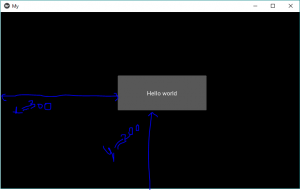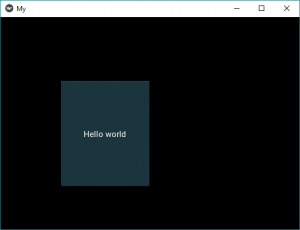# Python | Float Layout in Kivy

Kivy is a platform independent GUI tool in Python. As it can be run on Android, IOS, linux and Windows etc. It is basically used to develop the Android application, but it does not mean that it can not be used on Desktops applications.

### FloatLayout:

`Floatlayout `allows us to place the elements relatively based on the current window size and height especially in mobiles i.e. `Floatlayout `allow us to place the elements using something called relative position. This means rather than defining the specific position or the coordinates we will place everything using the percentage w.r.t the size of window. When we change the dimensions of the window everything placed in the window will adjust its size and position accordingly. This makes the Application more reliable and scaleable to window size.

Note: FloatLayout honors the pos_hint and the size_hint properties of its children.

The first thing we need to do to use a FloatLayout is import it.

`from kivy.uix.floatlayout import FloatLayout`
```Basic Approach:

1) import kivy
2) import kivyApp
3) import button
4) import Floatlayout
5) Set minimum version(optional)
6) create App class
7) return Layout/widget/Class(according to requirement)
8) Run an instance of the class```

For example, a FloatLayout with a size of (300, 300) is created:

`layout = FloatLayout(size=(300, 300))`

By default, all widgets have their `size_hint = (1, 1)`, so this below button will adopt the same size as the layout:

```button = Button(text='Hello world')

To create a button having a specific width and height of layout placed at a particular position you can do like below –

Implementation of the Approach:

 `# Sample Python application demonstrating the ` `# working of FloatLayout in Kivy ` ` `  `import` `kivy ` ` `  `# base Class of your App inherits from the App class.   ` `# app:always refers to the instance of your application   ` `from` `kivy.app ``import` `App ` ` `  `# creates the button in kivy  ` `# if not imported shows the error  ` `from` `kivy.uix.button ``import` `Button ` ` `  `# module consist the floatlayout ` `# to work with FloatLayout first ` `# you have to import it ` `from` `kivy.uix.floatlayout ``import` `FloatLayout ` ` `  `# To change the kivy default settings  ` `# we use this module config  ` `from` `kivy.config ``import` `Config  ` `   `  `# 0 being off 1 being on as in true / false  ` `# you can use 0 or 1 && True or False  ` `Config.``set``(``'graphics'``, ``'resizable'``, ``True``)  ` ` `  `# creating the App class ` `class` `MyApp(App): ` ` `  `    ``def` `build(``self``): ` ` `  `        ``# creating Floatlayout ` `        ``Fl ``=` `FloatLayout() ` ` `  `        ``# creating button ` `        ``# a button 30 % of the width and 20 % ` `        ``# of the height of the layout and ` `        ``# positioned at (300, 200), you can do: ` `        ``btn ``=` `Button(text ``=``'Hello world'``, ` `                    ``size_hint ``=``(.``3``, .``2``), ` `                    ``pos ``=``(``300``, ``200``)) ` ` `  `        ``# adding widget i.e button ` `        ``Fl.add_widget(btn) ` ` `  `        ``# return the layout ` `        ``return` `Fl ` ` `  `# run the App ` `if` `__name__ ``=``=` `"__main__"``: ` `    ``MyApp().run() `

Output:Note: Now if you change the size of window, it changes its position relatively and size also. This layout can be used for an application. Most of the time, you will use the size of the Window.

Dynamic Placements –
Now there is something missing or the above code is not perfect you can say. We still need to add the placement for the buttons.

We have 2 properties to create dynamic placement:

1) pos_hint: provide hint of position
We can define upto 6 keys i.e. it takes arguments in form of dictionary.
pos_hint = {“x”:1, “y”:1, “left”:1, “right”:1, “top”:1, “bottom”:1}

2) size_hint: provide hint of size
Contains two arguments i.e. width and height

Note:

1. You can only use values between 0-1 for both size_hint and pos_hint. Where 0 = 0% and 1 = 100%.
2. The coordinate system in kivy works from the bottom left! This will be important when placing our objects. (i.e (0, 0) is the bottom left).

Code to implement dynamic positioning:

 `# Sample Python application demonstrating the ` `# working of Dynamic placement in FloatLayout in Kivy ` ` `  `import` `kivy ` ` `  `# base Class of your App inherits from the App class.   ` `# app:always refers to the instance of your application   ` `from` `kivy.app ``import` `App ` ` `  `# creates the button in kivy  ` `# if not imported shows the error  ` `from` `kivy.uix.button ``import` `Button ` ` `  `# module consist the floatlayout ` `# to work with FloatLayout first ` `# you have to import it ` `from` `kivy.uix.floatlayout ``import` `FloatLayout ` ` `  `# To change the kivy default settings  ` `# we use this module config  ` `from` `kivy.config ``import` `Config  ` `   `  `# 0 being off 1 being on as in true / false  ` `# you can use 0 or 1 && True or False  ` `Config.``set``(``'graphics'``, ``'resizable'``, ``True``)  ` ` `  `# creating the App class ` `class` `MyApp(App): ` ` `  `    ``def` `build(``self``): ` ` `  `        ``# creating Floatlayout ` `        ``Fl ``=` `FloatLayout() ` ` `  `        ``# creating button ` `        ``# a button 30 % of the width and 50 % ` `        ``# of the height of the layout and ` `        ``# positioned at 20 % right and 20 % up ` `        ``# from bottom left, i.e x, y = 200, 200 from bottom left: ` `        ``btn ``=` `Button(text ``=``'Hello world'``, size_hint ``=``(.``3``, .``5``), ` `                     ``background_color ``=``(.``3``, .``6``, .``7``, ``1``), ` `                    ``pos_hint ``=``{``'x'``:.``2``, ``'y'``:.``2` `}) ` ` `  `        ``# adding widget i.e button ` `        ``Fl.add_widget(btn) ` ` `  `        ``# return the layout ` `        ``return` `Fl ` ` `  `# run the App ` `if` `__name__ ``=``=` `"__main__"``: ` `    ``MyApp().run() `

Output:Video Output:

Reference:
https://kivy.org/doc/stable/api-kivy.uix.floatlayout.html
https://techwithtim.net/tutorials/kivy-tutorial/floatlayout/

My Personal Notes arrow_drop_upCheck out this Author's contributed articles.

If you like GeeksforGeeks and would like to contribute, you can also write an article using contribute.geeksforgeeks.org or mail your article to contribute@geeksforgeeks.org. See your article appearing on the GeeksforGeeks main page and help other Geeks.

Please Improve this article if you find anything incorrect by clicking on the "Improve Article" button below.

Improved By : ManasChhabra2, nidhi_biet

Article Tags :

Be the First to upvote.

Please write to us at contribute@geeksforgeeks.org to report any issue with the above content.